Tamil Nadu Board of Secondary EducationTamil Nadu Primary School Class 4th
Advertisement Remove all ads

# Samacheer Kalvi solutions for Mathematics Term 1 - Class 4th Tamil Nadu State Board chapter 1 - Geometry [Latest edition]

Advertisement Remove all ads

#### Chapters## Chapter 1: Geometry

Exercise 1.1Exercise 1.2Exercise 1.3Exercise 1.4Exercise 1.5Exercise 1.6Exercise 1.7
Exercise 1.1 [Page 3]

### Samacheer Kalvi solutions for Mathematics Term 1 - Class 4th Tamil Nadu State Board Chapter 1 Geometry Exercise 1.1 [Page 3]

Exercise 1.1 | Q A. (i) | Page 3

Write the name of shape in the following picture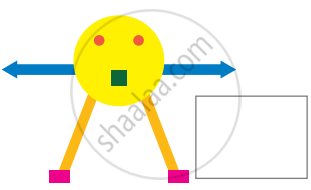Exercise 1.1 | Q A. (ii) | Page 3

Write the name of shape in the following picture.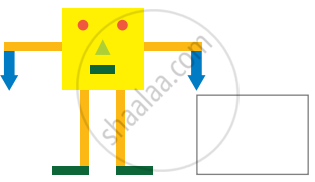Exercise 1.1 | Q B. | Page 3
 i Write the number of squares and triangles in the given picture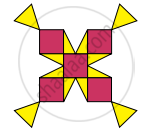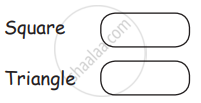ii Write the number of rectangles and triangles in the given picture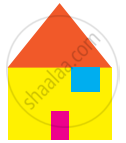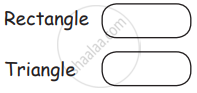iii Identify the shapes and write the names in the boxes given below.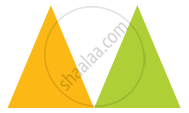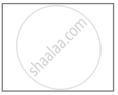Advertisement Remove all ads
Exercise 1.2 [Page 6]

### Samacheer Kalvi solutions for Mathematics Term 1 - Class 4th Tamil Nadu State Board Chapter 1 Geometry Exercise 1.2 [Page 6]

#### Fill in the blanks

Exercise 1.2 | Q A. i. | Page 6

All closed four sided figure are called ______________.

Exercise 1.2 | Q A. ii. | Page 6

A ______________ has four equal sides and equal diagonals

Exercise 1.2 | Q A. iii. | Page 6

The opposite sides of a ______________ are equal.

Exercise 1.2 | Q A. iv. | Page 6

A _____________ has no sides.

Exercise 1.2 | Q A. v. | Page 6

Diagonals are equal in ____________ and ____________.

Exercise 1.2 | Q B. | Page 6

Write the name of the sides and diagonals.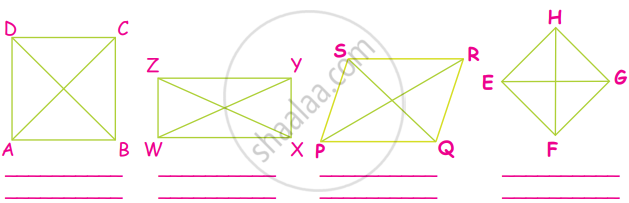Advertisement Remove all ads
Exercise 1.3 [Page 7]

### Samacheer Kalvi solutions for Mathematics Term 1 - Class 4th Tamil Nadu State Board Chapter 1 Geometry Exercise 1.3 [Page 7]

Exercise 1.3 | Q a. | Page 7

Draw circle for the following measurement.

6 cm

Exercise 1.3 | Q b. | Page 7

Draw circle for the following measurement.

5.5 cm

Exercise 1.3 | Q c. | Page 7

Draw circle for the following measurement.

8 cm

Exercise 1.3 | Q d. | Page 7

Draw circle for the following measurement.

6.8 cm

Exercise 1.3 | Q e. | Page 7

Draw circle for the following measurement.

8.6 cm

Advertisement Remove all ads
Exercise 1.4 [Pages 8 - 9]

### Samacheer Kalvi solutions for Mathematics Term 1 - Class 4th Tamil Nadu State Board Chapter 1 Geometry Exercise 1.4 [Pages 8 - 9]

#### Fill in the blanks

Exercise 1.4 | Q A. i. | Page 8

All the radii of a circle are _______________

Exercise 1.4 | Q A. ii. | Page 8

The ______________ is the longest chord of a circle

Exercise 1.4 | Q A. iii. | Page 8

A line segment joining any point on the circle to its center is called the _____________ of the circle

Exercise 1.4 | Q A. iv. | Page 8

A line segment with its end points on the circle is called a ______________

Exercise 1.4 | Q A. v. | Page 8

Twice the radius is ________________

Exercise 1.4 | Q B. i. | Page 9

Find the diameter of the circle

Radius = 10 cm

Exercise 1.4 | Q B. ii. | Page 9

Find the diameter of the circle

Radius = 8 cm

Exercise 1.4 | Q B. iii. | Page 9

Find the diameter of the circle

Radius = 6 cm

Exercise 1.4 | Q C. i. | Page 9

Find the radius of the circle

Diameter = 24 cm

Exercise 1.4 | Q C. ii. | Page 9

Find the radius of the circle

Diameter = 30 cm

Exercise 1.4 | Q C. iii. | Page 9

Find the radius of the circle

Diameter = 76 cm

Advertisement Remove all ads
Exercise 1.5 [Page 11]

### Samacheer Kalvi solutions for Mathematics Term 1 - Class 4th Tamil Nadu State Board Chapter 1 Geometry Exercise 1.5 [Page 11]

Exercise 1.5 | Q A. i. | Page 11

Find the perimeter of the following figure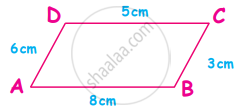Exercise 1.5 | Q A. ii. | Page 11

Find the perimeter of the following figure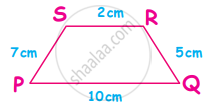Exercise 1.5 | Q A. iii. | Page 11

Find the perimeter of the following figure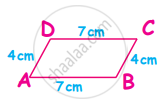Exercise 1.5 | Q A. iv. | Page 11

Find the perimeter of the following figure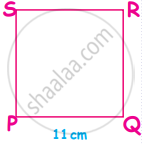Exercise 1.5 | Q A. v. | Page 11

Find the perimeter of the following figure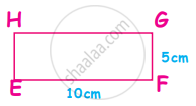#### Solve the following.

Exercise 1.5 | Q B. 1. | Page 11

A side of a square-shaped sandbox in Gandhi Park measures 30 cm. Determine the perimeter of the sandbox

Exercise 1.5 | Q B. 2. | Page 11

Find the perimeter of a rectangle, whose sides are 12 cm and 8 cm

Exercise 1.5 | Q B. 3. | Page 11

Find the perimeter of the triangle, whose sides are 13 cm, 5 cm and 14 cm

Exercise 1.5 | Q B. 4. | Page 11

The adjacent sides of a parallelogram are 6 cm and 7 cm. What is the perimeter of the parallelogram?

Exercise 1.5 | Q B. 5. | Page 11

The sides of a trapezoid measure 8 cm, 7 cm, 4 cm and 5 cm respectively. What is the perimeter of the trapezoid?

Advertisement Remove all ads
Exercise 1.6 [Page 14]

### Samacheer Kalvi solutions for Mathematics Term 1 - Class 4th Tamil Nadu State Board Chapter 1 Geometry Exercise 1.6 [Page 14]

Exercise 1.6 | Q A. i. | Page 14

Fill the following diagram with appropriate tile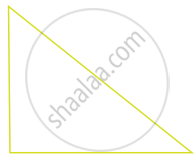Exercise 1.6 | Q A. ii. | Page 14

Fill the following diagram with appropriate tile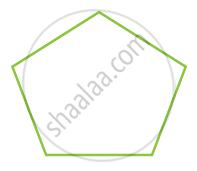Advertisement Remove all ads
Exercise 1.7 [Page 17]

### Samacheer Kalvi solutions for Mathematics Term 1 - Class 4th Tamil Nadu State Board Chapter 1 Geometry Exercise 1.7 [Page 17]

#### Choose the correct answer

Exercise 1.7 | Q A. i. | Page 17

A cuboid has ______________ edges

• 6

• 8

• 12

Exercise 1.7 | Q A. ii. | Page 17

The shape of a dice is like a _______________

• cuboid

• cube

• sphere

Exercise 1.7 | Q A. iii. | Page 17

A _____________ has a curved surface and two plane faces

• cylinder

• cone

• sphere

Exercise 1.7 | Q A. iv. | Page 17

I have one vertex and one plane face. I am a _______________

• cone

• cylinder

• sphere

Exercise 1.7 | Q A. v. | Page 17

A cube has _______________ vertices

• 8

• 12

• 6

Advertisement Remove all ads

## Chapter 1: Geometry

Exercise 1.1Exercise 1.2Exercise 1.3Exercise 1.4Exercise 1.5Exercise 1.6Exercise 1.7## Samacheer Kalvi solutions for Mathematics Term 1 - Class 4th Tamil Nadu State Board chapter 1 - Geometry

Samacheer Kalvi solutions for Mathematics Term 1 - Class 4th Tamil Nadu State Board chapter 1 (Geometry) include all questions with solution and detail explanation. This will clear students doubts about any question and improve application skills while preparing for board exams. The detailed, step-by-step solutions will help you understand the concepts better and clear your confusions, if any. Shaalaa.com has the Tamil Nadu Board of Secondary Education Mathematics Term 1 - Class 4th Tamil Nadu State Board solutions in a manner that help students grasp basic concepts better and faster.

Further, we at Shaalaa.com provide such solutions so that students can prepare for written exams. Samacheer Kalvi textbook solutions can be a core help for self-study and acts as a perfect self-help guidance for students.

Concepts covered in Mathematics Term 1 - Class 4th Tamil Nadu State Board chapter 1 Geometry are Two Dimensional Shapes, How to Draw a Circle Using a Compass, Concept of Circle - Centre, Radius, Diameter, Arc, Sector, Chord, Segment, Semicircle, Circumference, Interior and Exterior, Concentric Circles, Perimeter of a Rectangle, Perimeter of Squares, Perimeter of Triangles, Perimeter of Polygon, Creating Objects by Combining Different 2d Shapes, Three Dimensional Shapes.

Using Samacheer Kalvi Class 4th solutions Geometry exercise by students are an easy way to prepare for the exams, as they involve solutions arranged chapter-wise also page wise. The questions involved in Samacheer Kalvi Solutions are important questions that can be asked in the final exam. Maximum students of Tamil Nadu Board of Secondary Education Class 4th prefer Samacheer Kalvi Textbook Solutions to score more in exam.

Get the free view of chapter 1 Geometry Class 4th extra questions for Mathematics Term 1 - Class 4th Tamil Nadu State Board and can use Shaalaa.com to keep it handy for your exam preparation

Advertisement Remove all ads
Share
Notifications

View all notifications

Forgot password?